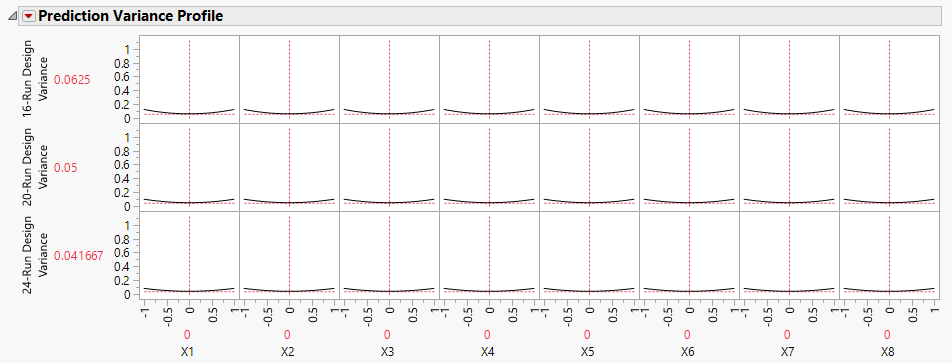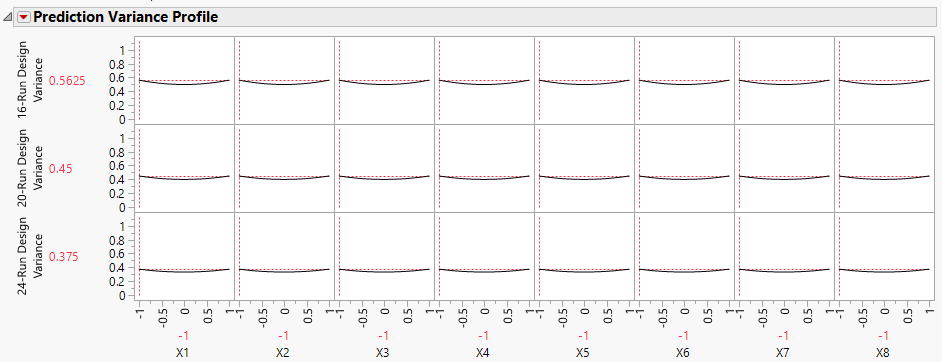Publication date: 04/12/2021

## Prediction Variance Profile

The Prediction Variance Profile outline shows profilers of the relative variance of prediction for each design being compared. Each plot shows the relative variance of prediction as a function of each factor at fixed values of the other factors.

To find the maximum value of the relative prediction variance over the design space for the reference design, select the Maximize Variance option from the red triangle next to Prediction Variance Profile. See Maximize Variance.

Figure 16.23 Prediction Variance Profile for Three DesignsThe Prediction Variance Profile plot in Figure 16.23 is for the three designs constructed in Designs of Different Run Sizes. Two two-way interactions, X1*X3 and X2*X3, have been added to the Model outline. The initial value for each continuous factor in the plot is the midpoint of its design settings. The Variance values to the left indicate that, as the number of runs increases, the variance decreases at the center point.

#### Relative Prediction Variance

For given settings of the factors, the prediction variance is the product of the error variance and a quantity that depends on the design and the factor settings. Before you run your experiment, the error variance is unknown, so the prediction variance is also unknown. However, the ratio of the prediction variance to the error variance is not a function of the error variance. This ratio, called the relative prediction variance, depends only on the design and the factor settings. Consequently, the relative variance of prediction can be calculated before acquiring the data. See Relative Prediction Variance.

After you run your experiment and fit a least squares model, you can estimate the error variance using the mean squared error (MSE) of the model fit. You can estimate the actual variance of prediction at any setting by multiplying the relative variance of prediction at that setting.

Ideally, the prediction variance is small throughout the design space. Generally, the error variance drops as the sample size increases. In comparing designs, a design with lower prediction variance on average is preferable.

#### Maximize Variance

You can also evaluate a design or compare designs in terms of the maximum relative prediction variance. Select the Maximize Variance option from the red triangle next to Prediction Variance Profile. JMP uses a desirability function that maximizes the relative prediction variance. The value of the Variance in the Prediction Variance Profile is the worst (least desirable from a design point of view) value of the relative prediction variance.

Figure 16.24 Prediction Variance Profile Showing Maximum Variance for Three DesignsFigure 16.24 shows the Prediction Variance Profile after Maximize Variance was selected for the three designs constructed in Designs of Different Run Sizes. As expected, the maximum relative prediction variance decreases as the run size increases. The plot also shows values of the factors that give this worst-case relative variance. However, keep in mind that many settings can lead to this same maximum relative variance.

Want more information? Have questions? Get answers in the JMP User Community (community.jmp.com).